### Home > GC > Chapter 8 > Lesson 8.3.3 > Problem8-112A

8-112A.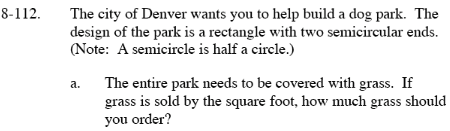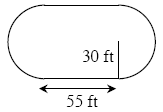Divide the park into three parts. Afterwards, find the area of each part then add them together.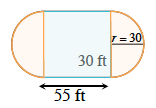Area of the rectangle = (l · w) = 55 · (2 · 30) = 3300 (where 2·30 is the diameter of the circle).

$\text{Area of one semicircle}=\frac{1}{2}\left ( \pi r^{2} \right )=450\pi$

Area of the dog park = semicircle + rectangle + semicircle = 450π + 3300 + 450π.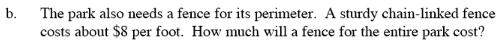Perimeter of the dog park = perimeter of semicircle + 55 + perimeter of semicircle + 55

$\text{Perimeter}=\frac{1}{2}2\pi r+55+\frac{1}{2}2\pi r+55$

After finding the perimeter, multiply your answer by 8 to determine the cost.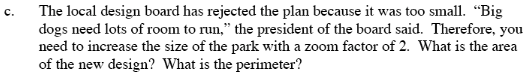The area of the new park = (the area of the old park)(the zoom factor)² ft
The perimeter of the new park = (the perimeter of the old park)(the zoom factor) ft